Next: 2.1.2 Pair-Diffusion Mechanism Up: 2.1 Diffusion Previous: 2.1 Diffusion

## 2.1.1 Extrinsic Dopant Diffusion

At usual diffusion temperatures the dopants are often ionized and the so released electrons cause an electric field which operates as an additional drift term to the normal diffusion flux.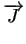= - D . grad (C) +. C (2.4)

The velocity of the charged particles can be described with=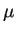.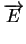= -. grad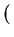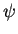(2.5)

where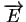is the electric field andthe electrostatic potential. The mobilityis related to the diffusion coefficient by Einstein's relation= D .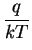(2.6)

where q denotes the elementary charge and k is known as the Boltzmann constant. Introducing (2.6) and (2.5) into (2.4) and taking more than one dopant into account Fick's law can be extended to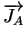= - DA .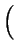grad (CA) + zA .. CA . grad ()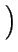(2.7)

where zA denotes the charge state of the belonging dopant (+1 for singly charged acceptors and -1 for singly charged donors).

The electrostatic potentialis determined by the Poisson equation

 div. grad ()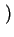= q . (n - p - Cnet) (2.8)

with
 Cnet = -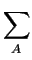zA . CA (2.9)

where the quantity Cnet represents the net concentration of all ionized dopants and n, p the concentration of electrons and holes, respectively.

Under the assumption of thermodynamic equilibrium the carrier concentrations n and p can be obtained with

 n . p = ni2 (2.10) n = ni . e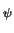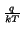(2.11) p = ni . e-(2.12)

In case of global charge neutrality

 n - p - Cnet = 0 (2.13)

and by means of the equilibrium carrier concentrations (2.11)(2.12) the electrostatic potential can explicitly be calculated by=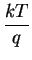. asinh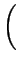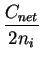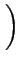(2.14)

With the explicit form of the gradient of,
 grad () = -.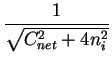.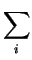zi . Ci (2.15)

substituting into (2.7) the flux for dopant CA now depends also on the gradients of the concentrations Ci of all other dopants.= - DA .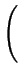1 +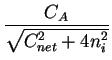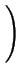. grad (CA) - DA ..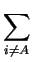zi . grad (Ci) (2.16)

If only one dopant is present (2.16) simplifies to= - DA .1 +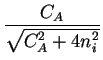. grad (CA) (2.17)

Comparing Fick's first law (2.1) and (2.17) an effective diffusion coefficient with the field enhancement factorcan be extracted

 Deff = DA .1 +=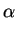. DA (2.18)

For intrinsic conditions ( CA/ni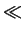1) this factor has a value close to one and for high concentrations ( CA/ni1) a value close to two.Next: 2.1.2 Pair-Diffusion Mechanism Up: 2.1 Diffusion Previous: 2.1 Diffusion